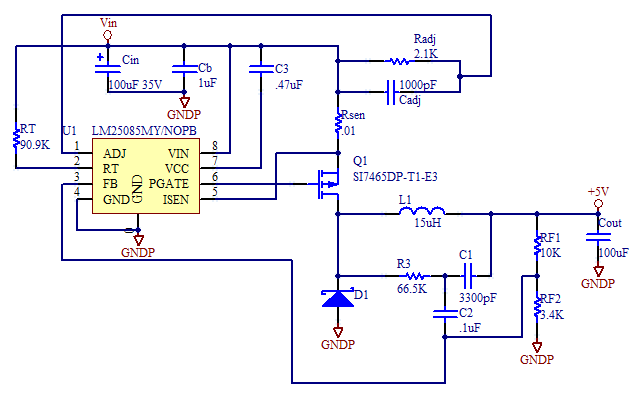# LM25085 Switching Regulator Design Calculator

The LM25085 is an affordable switching regulator in a small package, with a wide input voltage range. It's our experience that it's much better than the similar LM3489 which tends to run at too fast a frequency and overheat. The LM25085 has pad under it for better heat transfer. It also has very good design equations which are easy to follow, but tedious to implement by hand. This standard design uses the Vishay Si7465 P channel MOSFET, which has a low gate chare of only 40nC. The power disipation of the LM25085 is directly proportional to the gate charge of the MOSFET so this should be minimized.

R3 and C3 form a product. So you can scale them together. For example if you use a 0.01uF cap for C3 then divide the calculator's result for R3 by a factor 3.

### Troubleshooting

I had a problem where the regulator wouldn't start up if I used a power supply with a very fast rise time, or alternatively, if I just apply power to the input, with the power supply on. If I used a slow rise time power supply, it would start up just fine. I noticed, that with the fast rise time input voltage, it would pull RT low, indicating some sort of error state, and the output voltage of the regulator was essentially nill. The input voltage of my supply was 24V and and the output of the regulator was intended to be 12V.

I solved the problem by lower the value of the inductor. I speculate that the inductor was saturating, and tripping current protection inside the regulator, and so when I used a lower inductance inductor, from the same inductor series, the saturation current for the lower valued inductors is higher, than that of the higher valued inductors. This is just a guess.

### Schematic### Calculator

 Maximum Input Voltage (Vin(max)) (Volts) Nominal Input Voltage (Vin(nominal)) (Volts) Minimum Input Voltage (Vin(min)) (Volts) Output Voltage (Vout) (Volts) Diode Forward Voltage (Vd) (Volts) Maximum Output Current (Imax). (Amps) Minimum Output Current (Imin) (Amps) Maximum Output Voltage Ripple (mVpp) Current Sense Resistor. Choose .01 ohms if unsure. (ohms) Top Feedback Resistor Rf1 Choose 10 Kohms if unsure. (Kohms) Desired Switching Frequency (Fs) (KHz) MOSFET On Time (nano secs) MOSFET Off Time (nano secs) MOSFET Gate Charge (nC) Duty Cycle (%) Ton(min) (Minimum Switch On Time) (nano secs) Ton(max) (Maximum Switch On Time) (nano secs) Maximum Diode Power (W) Maximum LM25085 Power (W) Maximum Current Sense Resistor Power (W) Inductor (L) (uH) Current Limit (Icl).(Peak Inductor Current) (Amps) Output Capacitor, (Cout) (uF) Input Capacitor, (Cin) (uF) Rf2 (Kohms) RT (Kohms) Radj (ohms) Cadj (pf) R3*C1 Product C1 (pf) R3 (Kohms) C2 (uf)

[Plant Database], [Soil Moisture Sensor] [Water Level Sensor] [Soil Moisture Meter]